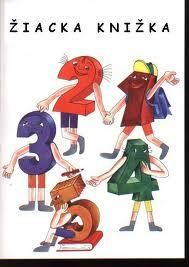# Students 5905

There were 21 pupils in the class, and the teacher gave the mark one to 3 pupils, mark two to 5 pupils, mark three to 8 pupils, mark four to 4 pupils, and five to 1 pupils. How many percent of students received a grade better than three?

p =  38.0952 %

### Step-by-step explanation:Did you find an error or inaccuracy? Feel free to write us. Thank you!

Tips for related online calculators
Looking for help with calculating arithmetic mean?
Looking for a statistical calculator?
Our percentage calculator will help you quickly calculate various typical tasks with percentages.

#### Grade of the word problem:

We encourage you to watch this tutorial video on this math problem: